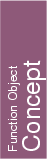## Kernel::ConstructIsoCuboid_3

A model for this must provide:

Kernel::Iso_cuboid_3
 fo.operator() ( Kernel::Point_3 p, Kernel::Point_3 q)
introduces an iso-oriented cuboid with diagonal opposite vertices p and q such that p is the lexicographically smallest point in the cuboid.

Kernel::Iso_cuboid_3
 fo.operator() ( Kernel::Point_3 left, Kernel::Point_3 right, Kernel::Point_3 bottom, Kernel::Point_3 top, Kernel::Point_3 far, Kernel::Point_3 close)
introduces an iso-oriented cuboid fo whose minimal x coordinate is the one of left, the maximal x coordinate is the one of right, the minimal y coordinate is the one of bottom, the maximal y coordinate is the one of top, the minimal z coordinate is the one of far, the maximal z coordinate is the one of close.